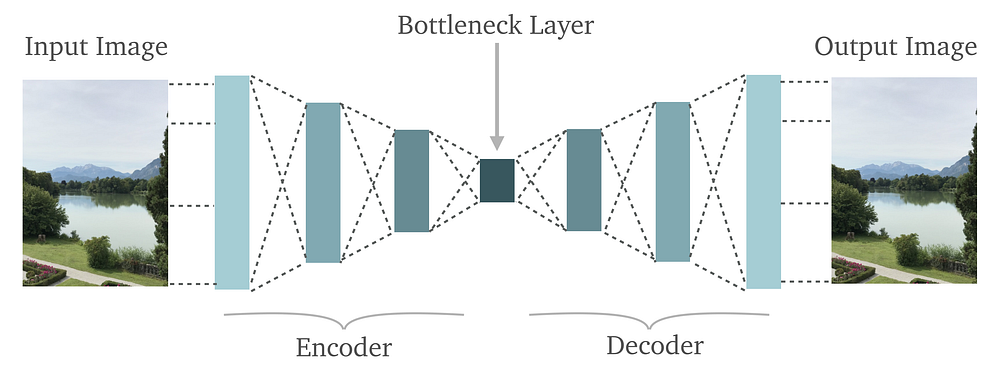# Autoencoders: Unsupervised Artificial Neural Networks(ANN)

Original article was published on Deep Learning on MediumThis image was extracted from the G. E. Hinton and R. R. Salakhutdinovcomparing’s paper, on the two-dimensional reduction for 500 digits of the MNIST, with PCA on the left and autoencoder on the right. We can see that the autoencoder provided us with a better separation of data.

# 5. Code

`#from __future__ import division, print_function, absolute_importimport tensorflow as tfimport numpy as npimport matplotlib.pyplot as plt%matplotlib inline# Import MINST datafrom tensorflow.examples.tutorials.mnist import input_datamnist = input_data.read_data_sets(“/tmp/data/”, one_hot=True)`

Now, let’s give the parameters that are going to be used by our NN.

`learning_rate = 0.01training_epochs = 20batch_size = 256display_step = 1examples_to_show = 10# Network Parametersn_hidden_1 = 256 # 1st layer num featuresn_hidden_2 = 128 # 2nd layer num featuresn_input = 784 # MNIST data input (img shape: 28*28)# tf Graph input (only pictures)X = tf.placeholder(“float”, [None, n_input])weights = { ‘encoder_h1’: tf.Variable(tf.random_normal([n_input, n_hidden_1])), ‘encoder_h2’: tf.Variable(tf.random_normal([n_hidden_1, n_hidden_2])), ‘decoder_h1’: tf.Variable(tf.random_normal([n_hidden_2, n_hidden_1])), ‘decoder_h2’: tf.Variable(tf.random_normal([n_hidden_1, n_input])),}biases = { ‘encoder_b1’: tf.Variable(tf.random_normal([n_hidden_1])), ‘encoder_b2’: tf.Variable(tf.random_normal([n_hidden_2])), ‘decoder_b1’: tf.Variable(tf.random_normal([n_hidden_1])), ‘decoder_b2’: tf.Variable(tf.random_normal([n_input])),}`

Now we need to create our encoder. For this, we are going to use sigmoidal functions. Sigmoidal functions deliver great results with this type of network. This is due to having a good derivative that is well-suited to backpropagation. We can create our encoder using the sigmoidal function like this:

`# Building the Encoderdef encoder(x): # Encoder first layer with sigmoid activation #1 layer_1 = tf.nn.sigmoid(tf.add(tf.matmul(x, weights['encoder_h1']), biases['encoder_b1'])) # Encoder second layer with sigmoid activation #2 layer_2 = tf.nn.sigmoid(tf.add(tf.matmul(layer_1, weights['encoder_h2']), biases['encoder_b2'])) return layer_2`

And the decoder: You can see that the layer_1 in the encoder is the layer_2 in the decoder and vice-versa.

`# Building the decoderdef decoder(x): # Decoder first layer with sigmoid activation #1 layer_1 = tf.nn.sigmoid(tf.add(tf.matmul(x, weights['decoder_h1']),biases['decoder_b1'])) # Decoder second layer with sigmoid activation #2 layer_2 = tf.nn.sigmoid(tf.add(tf.matmul(layer_1, weights['decoder_h2']), biases['decoder_b2'])) return layer_2`

Let’s construct our model. In the variable `cost` we have the loss function and in the `optimizer` variable we have our gradient used for backpropagation.

`# Launch the graph# Using InteractiveSession (more convenient while using Notebooks)sess = tf.InteractiveSession()sess.run(init)total_batch = int(mnist.train.num_examples / batch_size)# Training cyclefor epoch in range(training_epochs): # Loop over all batches for i in range(total_batch): batch_xs, batch_ys = mnist.train.next_batch(batch_size) # Run optimization op (backprop) and cost op (to get loss value) _, c = sess.run([optimizer, cost], feed_dict={X: batch_xs}) # Display logs per epoch step if epoch % display_step == 0: print(“Epoch:”, ‘%04d’ % (epoch+1), “cost=”, “{:.9f}”.format(c))print(“Optimization Finished!”)`

Output:

`Epoch: 0001 cost= 0.182728916Epoch: 0002 cost= 0.150434598Epoch: 0003 cost= 0.130958572Epoch: 0004 cost= 0.125098571Epoch: 0005 cost= 0.119374141Epoch: 0006 cost= 0.116029739Epoch: 0007 cost= 0.114480294Epoch: 0008 cost= 0.110542893Epoch: 0009 cost= 0.107315414Epoch: 0010 cost= 0.103023507Epoch: 0011 cost= 0.101529025Epoch: 0012 cost= 0.097410828Epoch: 0013 cost= 0.093311585Epoch: 0014 cost= 0.093811013Epoch: 0015 cost= 0.090760238Epoch: 0016 cost= 0.089178301Epoch: 0017 cost= 0.087290406Epoch: 0018 cost= 0.085913278Epoch: 0019 cost= 0.086014777Epoch: 0020 cost= 0.084903874Optimization Finished!`

Above we have taken 20 Epochs.

Now, let’s apply encoder and decoder for our tests.

`# Applying encode and decode over test setencode_decode = sess.run( y_pred, feed_dict={X: mnist.test.images[:examples_to_show]})# Lets Let’s simply visualize our graphs!# Compare original images with their reconstructionsf, a = plt.subplots(2, 10, figsize=(10, 2))for i in range(examples_to_show): a[i].imshow(np.reshape(mnist.test.images[i], (28, 28))) a[i].imshow(np.reshape(encode_decode[i], (28, 28)))`